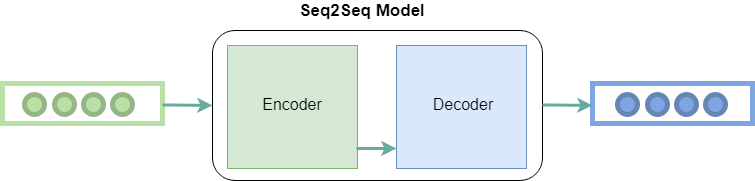# seq2seq Model in Machine Learning

Attention is all you need! was a research paper that first introduced the transformer model in the era of Deep Learning after which language-related models have taken a huge leap. The main idea behind the transformers model was that of attention layers and different encoder and decoder stacks which were highly efficient to perform language-related tasks.

## What is seq2seq Model in Machine Learning?

Seq2seq was first introduced for machine translation, by Google. Before that, the translation worked in a very naïve way. Each word that you used to type was converted to its target language giving no regard to its grammar and sentence structure. Seq2seq revolutionized the process of translation by making use of deep learning. It not only takes the current word/input into account while translating but also its neighborhood.

Seq2Seq (Sequence-to-Sequence) is a type of model in machine learning that is used for tasks such as machine translation, text summarization, and image captioning. The model consists of two main components:

• Encoder
• Decoder

Seq2Seq models are trained using a dataset of input-output pairs, where the input is a sequence of tokens and the output is also a sequence of tokens. The model is trained to maximize the likelihood of the correct output sequence given the input sequence.

Seq2Seq models have been widely used in NLP tasks such as machine translation, text summarization, and image captioning, due to their ability to handle variable-length input and output sequences. Additionally, the Attention mechanism is often used in Seq2Seq models to improve performance and it allows the decoder to focus on specific parts of the input sequence when generating the output.

Nowadays, it is used for a variety of different applications such as image captioning, conversational models, text summarization, etc.

## Encoder-Decoder Stack

As the name suggests, seq2seq takes as input a sequence of words(sentence or sentences) and generates an output sequence of words. It does so by use of the recurrent neural network (RNN). Although the vanilla version of RNN is rarely used, its more advanced version i.e. LSTM or GRU is used. This is because RNN suffers from the problem of vanishing gradient. LSTM is used in the version proposed by Google. It develops the context of the word by taking 2 inputs at each point in time. One from the user and the other from its previous output, hence the name recurrent (output goes as input).

The encoder and decoder are typically implemented as Recurrent Neural Networks (RNNs) or Transformers.

### Encoder Stack

It uses deep neural network layers and converts the input words to corresponding hidden vectors. Each vector represents the current word and the context of the word. The encoder takes the input sequence, one token at a time, and uses an RNN or transformer to update its hidden state, which summarizes the information in the input sequence. The final hidden state of the encoder is then passed as the context vector to the decoder.

### Decoder Stack

It is similar to the encoder. It takes as input the hidden vector generated by the encoder, its own hidden states, and the current word to produce the next hidden vector and finally predict the next word. The decoder uses the context vector and an initial hidden state to generate the output sequence, one token at a time. At each time step, the decoder uses the current hidden state, the context vector, and the previous output token to generate a probability distribution over the possible next tokens. The token with the highest probability is then chosen as the output, and the process continues until the end of the output sequence is reached.Encoder and Decoder Stack in seq2seq model

## Components of seq2seq Model in Machine Learning

Apart from these two, many optimizations have led to other components of seq2seq:

• Attention: The input to the decoder is a single vector that has to store all the information about the context. This becomes a problem with large sequences. Hence the attention mechanism is applied which allows the decoder to look at the input sequence selectively.
• Beam Search: The highest probability word is selected as the output by the decoder. But this does not always yield the best results, because of the basic problem of greedy algorithms. Hence beam search is applied which suggests possible translations at each step. This is done by making a tree of top k-results.
• Bucketing: Variable-length sequences are possible in a seq2seq model because of the padding of 0’s which is done to both input and output. However, if the max length set by us is 100 and the sentence is just 3 words long it causes a huge waste of space. So we use the concept of bucketing. We make buckets of different sizes like (4, 8) (8, 15), and so on, where 4 is the max input length defined by us and 8 is the max output length defined.

• Flexibility: Seq2Seq models can handle a wide range of tasks such as machine translation, text summarization, and image captioning, as well as variable-length input and output sequences.
• Handling Sequential Data: Seq2Seq models are well-suited for tasks that involve sequential data such as natural language, speech, and time series data.
• Handling Context: The encoder-decoder architecture of Seq2Seq models allows the model to capture the context of the input sequence and use it to generate the output sequence.
• Attention Mechanism: Using attention mechanisms allows the model to focus on specific parts of the input sequence when generating the output, which can improve performance for long input sequences.